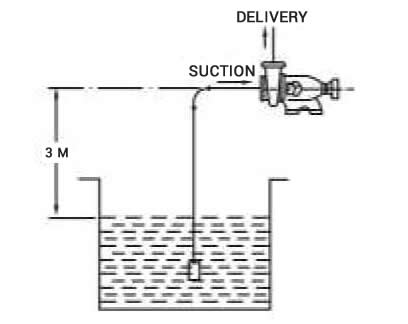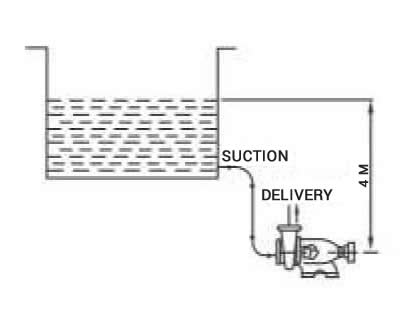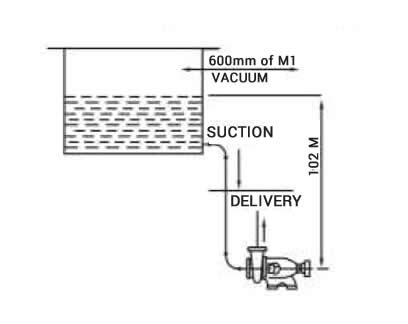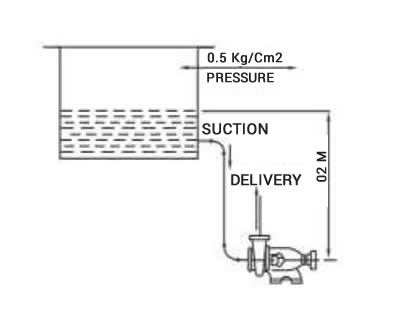# NPSH Calculation

###### Home  |  NPSH Calculation

NPSH (A) = (Pressure on the liquid surface) (ha) ± (suction head/lift) (friction loss) (vapour pressure) (hvp)

### EXAMPLE – I OPEN TANK – SUCTION LIFT CONDITIONDATA:
Source Pressure = 1 Kg / Cm2
Suction Lift – 3 m
Friction loss in pipeline
Foot Valve, Strainer, Bend, Valve
1m, temperature of water : 21°C

METHOD:
ha = 10 x Pa / Sg = 10 x 1 / 0.998 = 10.02 Mts
Vapour pressure of water at 21°C = 0.0253 Kg / Cm2
hvp = 10 x 0.0253 / 0.998 = 0.25 m
NPSH (A) = 10.02 – 3 – 1 – 0.25 = 5.77 mts

DATA:
Suction head: 4 m, Friction loss :1.2 m
Temperature : 21°C Attitude : 1500m
Pressure at 1500m Attitude is 0.9 kg / cm2
So, ha = 10 x 0.9 / 0.998 = 9.02 m
NPSH (A) = 9.02 + 4-1.2 – 0.25 = 11.57 Mts.

### EXAMPLE II OPEN TANK – SUCTION HEAD CONDITION### EXAMPLE – III CLOSED TANK UNDER VACCUM CONDITIONDATA:
Vaccum in Vessel 600 mm of HG
Temperature : 40°C, Friction Loss : 1m
Vapour pressure and specific gravity are 0.49 Kg / Cm2
and 0.72 respectively at 40°C. Sg of mercury : 13.6
ha = (10 x 1 / 0.72 – 600 x 13.6 / 1000 x 0.72)
= 13.88 – 11.33 = 2.55
hvp = 10 x 0.49 / 0.72 = 6.81 mts
NSH (A) = 2.55 + 10.2 -1 – 6.81 = 4.94 mts

DATA:
Pressure in closed vessel : 0.5 Kg / Cm2
Atomospheric Pressure at the installation : 0.9 Kg / cm2
Friction loss : 1.5 m, vapour pressure 0.45 Kg / Cm2
Specific gravity 0.8

METHOD:
ha = (10 x 0.9 / 0.8 + 10 x 0.5 / 0.8) = 11.25 + 6.25
= 17.5
hvp = ( 10 x 0.45 / 0.8) = 5.625
NPSH (A) = 17.25 + 0.2 – 5.625 – 1.5 = 10.325 mts

### EXAMPLE – III CLOSED TANK UNDER PRESSURE DATA